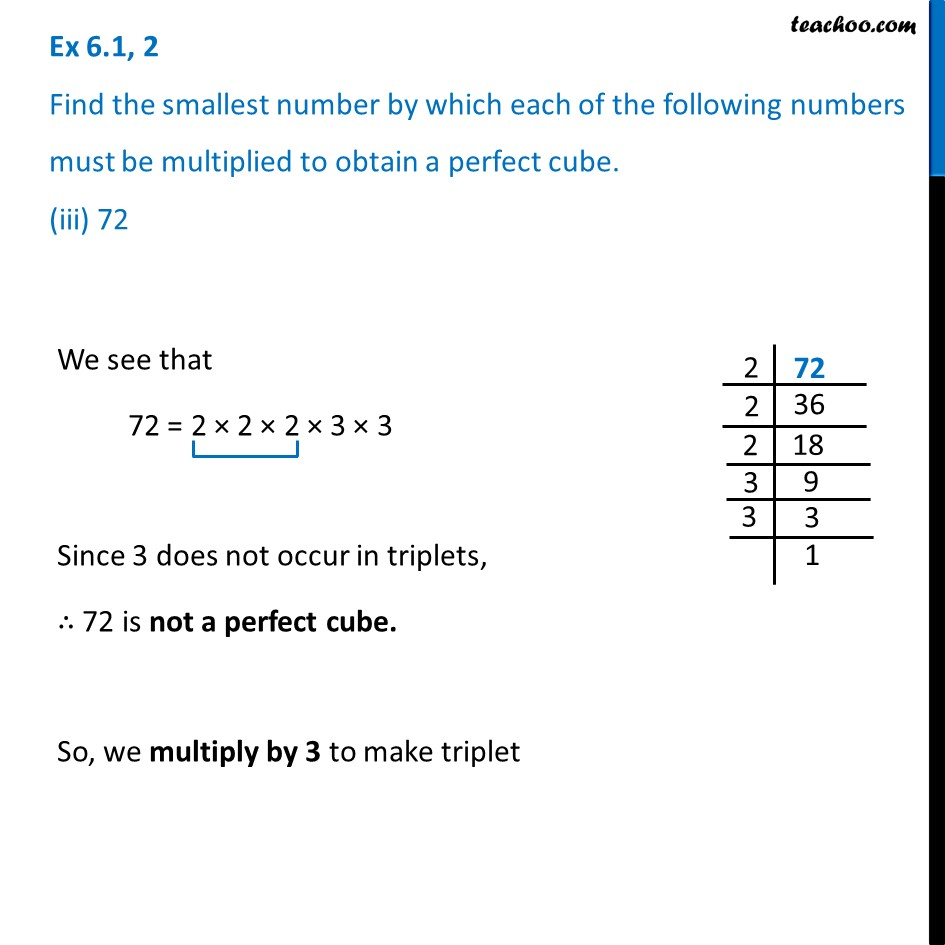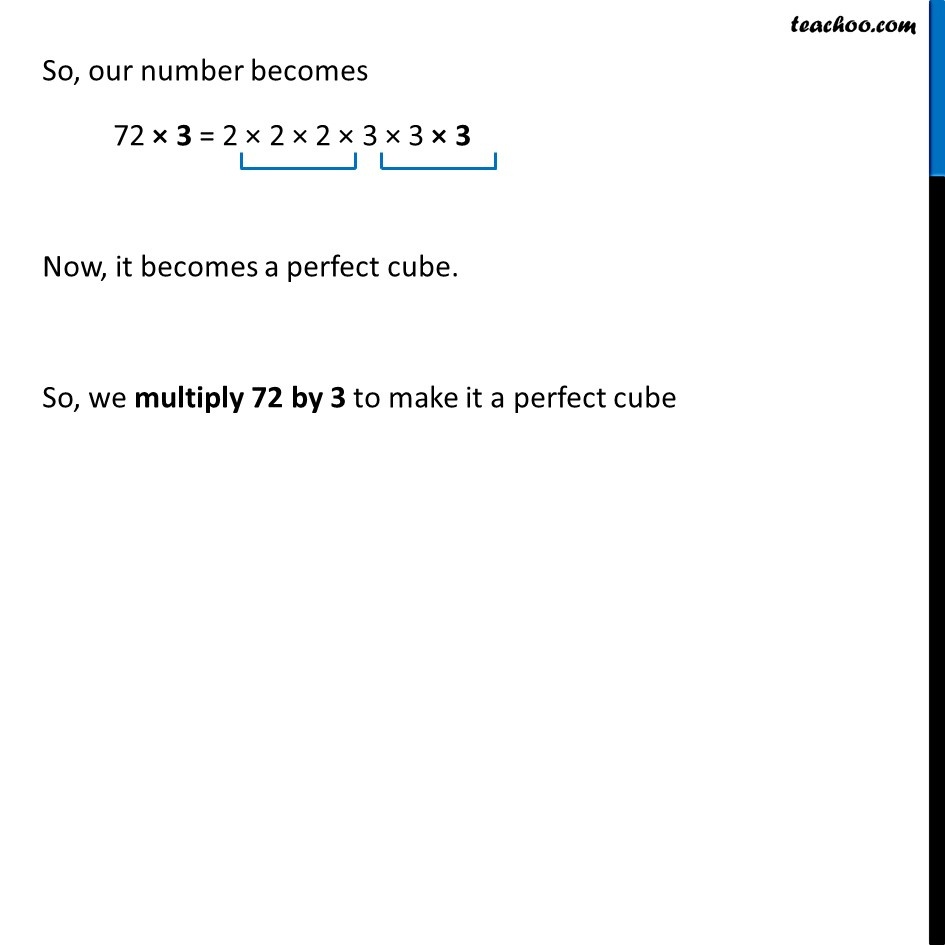Ex 6.1

Chapter 6 Class 8 Cubes and Cube Roots
Serial order wiseLearn in your speed, with individual attention - Teachoo Maths 1-on-1 Class

### Transcript

Ex 6.1, 2 Find the smallest number by which each of the following numbers must be multiplied to obtain a perfect cube. (iii) 72 We see that 72 = 2 × 2 × 2 × 3 × 3 Since 3 does not occur in triplets, ∴ 72 is not a perfect cube. So, we multiply by 3 to make triplet So, our number becomes 72 × 3 = 2 × 2 × 2 × 3 × 3 × 3 Now, it becomes a perfect cube. So, we multiply 72 by 3 to make it a perfect cube